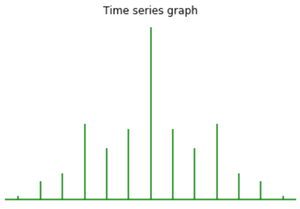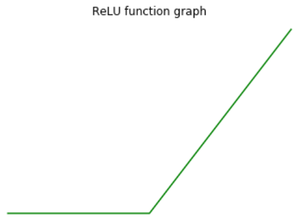Related Articles
How to Turn Off the Axes for Subplots in Matplotlib?
• Last Updated : 03 Jan, 2021

In this article, we are going to discuss how to turn off the axes of subplots using matplotlib module. We can turn off the axes for subplots and plots using the below methods:

Method 1: Using matplotlib.axes.Axes.axis()

To turn off the axes for subplots, we will matplotlib.axes.Axes.axis() method here.

## Python3

 `# import required modules``import` `matplotlib.pyplot as plt ``import` `matplotlib.tri as mtri ``import` `numpy as np ``     ` `# assign data    ``x ``=` `np.asarray([``0``, ``1``, ``2``, ``3``, ``0.5``, ``                ``1.5``, ``2.5``, ``1``, ``2``, ``                ``1.5``]) `` ` `y ``=` `np.asarray([``0``, ``0``, ``0``, ``0``, ``1.0``, ``                ``1.0``, ``1.0``, ``2``, ``2``, ``                ``3.0``]) `` ` `triangles ``=` `[[``0``, ``1``, ``4``], [``1``, ``5``, ``4``], ``            ``[``2``, ``6``, ``5``], [``4``, ``5``, ``7``], ``            ``[``5``, ``6``, ``8``], [``5``, ``8``, ``7``], ``            ``[``7``, ``8``, ``9``], [``1``, ``2``, ``5``], ``            ``[``2``, ``3``, ``6``]] `` ` `# depict illsutration``triang ``=` `mtri.Triangulation(x, y, triangles) ``z ``=` `np.cos(``1.5` `*` `x) ``*` `np.cos(``1.5` `*` `y) ``     ` `fig, axs ``=` `plt.subplots() ``axs.tricontourf(triang, z) ``axs.triplot(triang, ``'go-'``, color ``=``'white'``) `` ` `# turn off the axes``axs.set_axis_off()`` ` `# assign title``axs.set_title(``'Triangle illustration'``) `` ` `plt.show() `

Output:Here, we turn off axes using the axis(“off”) statement.

Method 2: Using matplotlib.axes.Axes.set_axis_off()

We use matplotlib.axes.Axes.set_axis_off() to turn the x-and y-pivot off influencing the axis lines, ticks, ticklabels, network and axis marks as well.

## Python3

 `# import required modules ``import` `matplotlib.pyplot as plt ``import` `numpy as np ``     ` `# time series data ``geeksx ``=` `np.array([``24.40``, ``110.25``, ``20.05``, ``                ``22.00``, ``61.90``, ``7.80``, ``                ``15.00``]) `` ` `geeksy ``=` `np.array([``24.40``, ``110.25``, ``20.05``, ``                ``22.00``, ``61.90``, ``7.80``, ``                ``15.00``]) ``     ` `# depict illustration    ``fig, ax ``=` `plt.subplots() ``ax.xcorr(geeksx, geeksy, maxlags ``=` `6``, ``        ``color ``=``"green"``) `` ` `# turn off the axes``ax.set_axis_off() `` ` `# assign title``ax.set_title(``'Time series graph'``) ``plt.show() `

Output:Method 3: Using matplotlib.pyplot.axis()

In a visualization, if the figure has a single plot in it, we can turn off the axes for subplots by making look like a contention to the matplotlib.pyplot.axis() technique. If the figure contains different subplots, this technique just turns off axes for the last subplot.

## Python3

 `# importing module``import` `matplotlib.pyplot as plt`` ` `# assigning x and y coordinates``x ``=` `[``-``5``, ``-``4``, ``-``3``, ``-``2``, ``-``1``, ``0``, ``1``, ``2``, ``3``, ``4``, ``5``]``y ``=` `[]`` ` `for` `i ``in` `range``(``len``(x)):``    ``y.append(``max``(``0``, x[i]))`` ` `# depicting the visualization``ax ``=` `plt.plot(x, y, color``=``'green'``)`` ` `# turn off the axes``plt.axis(``'off'``)`` ` `# displaying the title``plt.title(``"ReLU function graph"``)`` ` `plt.show()`

Output:Here, we turn off axes using the plt.axis(“off”) statement.

Attention geek! Strengthen your foundations with the Python Programming Foundation Course and learn the basics.

To begin with, your interview preparations Enhance your Data Structures concepts with the Python DS Course. And to begin with your Machine Learning Journey, join the Machine Learning – Basic Level Course

My Personal Notes arrow_drop_up